# JP2007010554A - Positioning device - Google Patents

## Info

Publication number
JP2007010554A
JP2007010554A JP2005193625A JP2005193625A JP2007010554A JP 2007010554 A JP2007010554 A JP 2007010554A JP 2005193625 A JP2005193625 A JP 2005193625A JP 2005193625 A JP2005193625 A JP 2005193625A JP 2007010554 A JP2007010554 A JP 2007010554A
Authority
JP
Japan
Prior art keywords
positioning
calculating
position
time constant
reliability
Prior art date
Legal status (The legal status is an assumption and is not a legal conclusion. Google has not performed a legal analysis and makes no representation as to the accuracy of the status listed.)
Pending
Application number
JP2005193625A
Other languages
Japanese (ja)
Inventor
Yasunori Aiga

Original Assignee

Priority date (The priority date is an assumption and is not a legal conclusion. Google has not performed a legal analysis and makes no representation as to the accuracy of the date listed.)
Filing date
Publication date
Application filed by Japan Radio Co Ltd, 日本無線株式会社 filed Critical Japan Radio Co Ltd
Priority to JP2005193625A priority Critical patent/JP2007010554A/en
Publication of JP2007010554A publication Critical patent/JP2007010554A/en
Application status is Pending legal-status Critical

## Images

•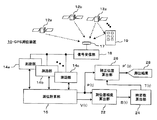•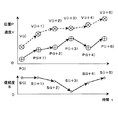•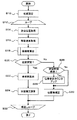•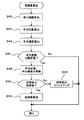•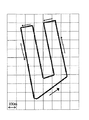•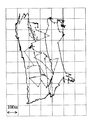•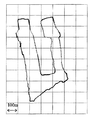## Abstract

<P>PROBLEM TO BE SOLVED: To smooth variation of a positioning result accompanying a movement and to achieve accuracy improvement of the positioning result. <P>SOLUTION: A positioning calculating section 16 of an on-vehicle GPS positioning device 10 outputs a position vector P(t) determined by a false range from a plurality of GPS satellites 12 and a velocity vector V(t) of the moving velocity calculated based on Doppler frequency to a positioning reliability calculating section 22. Next, the positioning reliability calculating section 22 determines reliability S(t), and outputs it to a time constant calculating section 24. The time constant calculating section 24 outputs time constant T(t) to a correction position calculating section 26. The correction position calculating section 26 acquires the position vector P(t) and time constant T(t), and outputs a corrected user position P'(t). <P>COPYRIGHT: (C)2007,JPO&INPIT

## Description

The present invention relates to a positioning device that performs positioning by receiving a signal transmitted by a satellite.

The satellite positioning system (GPS) has positioning means for receiving signals transmitted from a plurality of satellites and performing positioning calculation based on the orbit information of each satellite and the pseudo distance to each satellite from the information included in the received signal. It is a positioning device. In recent years, positioning devices using GPS have been widely used to detect the position of all objects such as mobile phones and vehicles.

When a mobile object equipped with a positioning device moves, the state of the GPS signal transmitted from the satellite changes and the GPS signal is interrupted by entering some shadow, or the GPS signal is some object (for example, a building) When a GPS signal is received by a positioning device through multiple paths (for example, multipath). When such GPS signal blocking or multipath occurs to the positioning device, the positioning result changes discontinuously, and a position jump phenomenon of the moving body position (hereinafter referred to as the user position) appears.

Therefore, Patent Document 1 discloses a technique for solving such a problem. In Patent Document 1, the position of the reception point obtained by the positioning means is smoothed by the movement distance at the predetermined time interval of the position and the movement distance at the predetermined time interval obtained from the Doppler frequency of the carrier of the GPS signal. Technology is shown. By using this technique, it is possible to provide a positioning device that smoothly outputs a change in the user position accompanying the movement of the reception point.

JP 2000-193732 A

However, the technique disclosed in Patent Document 1 has a problem that it only smoothes the change in the user position accompanying the movement and does not improve the accuracy of the positioning result.

Specifically, as the time constant T, which is an index of smoothing, is increased, the smoothing of the speed V (t) works more strongly and the effect of reducing the position P (t) error is greater. However, although the speed V (t) error is relatively small, if smoothing is continued, the small V (t) error is accumulated with time t, and the error is increased to the correction position P ′ (t) by smoothing. There is a concern that it will get worse and worse.

Thus, the effects of both errors are contradictory characteristics, and even if the time constant T is extended as compared with the current situation, it is difficult to sufficiently remove both errors and further improve the positioning accuracy. There is also a problem that the actual positioning result is delayed with smoothing.

In order to solve the problems as described above, a positioning device according to the present invention is mounted on a mobile body and receives a signal from a plurality of satellites, a code pseudo distance used for positioning from the received signals, and Positioning means for detecting the carrier Doppler frequency and the like to determine the position of the receiver, in the positioning device, the pseudo distance calculation means for calculating the pseudo distance from the satellite to the receiver, the receiver of the receiver from a plurality of pseudo distance Positioning calculation means for calculating position information, movement speed calculation means for calculating the moving speed of the receiver from the carrier Doppler frequency, and the correlation value between the calculated position information of the receiver and the moving speed as the reliability of the position information Positioning reliability calculation means for calculating, time constant calculating means for calculating a time constant that is inversely proportional to the calculated reliability as a time constant of position change, and time constant calculating means The time constant, and having a correction position calculating means for correcting the position information from the positioning calculating means.

Further, in the positioning device according to the present invention, the positioning reliability calculation means obtains a correlation value from the traveled position and the trajectory of the moving speed using the calculated position information and moving speed of the receiver, It is calculated as the reliability of the position information.

Further, the positioning device according to the present invention has integrated speed calculating means for calculating the integrated speed by integrating the moving speed from the moving speed calculating means, and the integrated speed calculating means is based on the time constant from the time constant calculating means. It is characterized in that an offset which is a difference between the position and the integrated speed is smoothed.

Furthermore, in the positioning device according to the present invention, the positioning reliability calculation means calculates the correlation value between the movement speed obtained from a sensor or the like that measures the motion state of the moving body and the position information from the positioning calculation means. It is calculated as the reliability of information.

The travel locus of the traveling position P (t) is essentially the same when compared with the travel locus of the speed V (t), but when there are many errors due to multipath, the locus of the position P (t). Is conspicuously different from the correct shape, and as a result, there is a feature that the difference between the trajectory shapes increases.

Therefore, in the positioning device according to the present invention, the positioning reliability calculation unit uses the position P (t) and the speed V (t) obtained by the positioning calculation unit to correlate the trajectory shapes of both of them that have traveled for a certain distance. The value is calculated as the reliability S (t) of the position P (t). Furthermore, the time constant setting unit determines a time constant T (t) that is inversely proportional to the reliability S (t).

By using the present invention, it is possible not only to smooth the change of the positioning result accompanying the movement, but also to improve the accuracy of the positioning result. For example, when it is determined that the error of the user position P (t) is large even if the time constant T is extended as compared with the conventional case, the time constant T (t) = T is set to be large so that the control is suitably performed. The Further, when it is determined that the error of the user position P (t) is almost zero, it is as small as T (t) ≈1, and the user velocity vector V at the correction position P ′ (t) = user position P (t). (T) Accumulation of errors can be prevented, and not only can the positioning accuracy of the correction position P ′ (t) be improved as compared to the conventional case, but also the positioning result delay can be improved.

Hereinafter, embodiments of the present invention (hereinafter referred to as embodiments) will be described with reference to the drawings.

FIG. 1 is a configuration diagram showing the overall configuration of a GPS positioning device 10 according to an embodiment of the present invention. The GPS positioning device 10 includes a GPS antenna 17, a signal receiving unit 18, a plurality of ranging units (14a, 14b, 14c), a positioning calculation unit 16, a positioning reliability calculation unit 22, and a time constant calculation unit 24. And a correction position calculation unit 26. A plurality of GPS satellites (12a, 12b, 12c) are arranged in the sky, and a signal reflected from the wall surface of the building 19 may be input to the GPS antenna 17 from time to time.

For example, the positioning calculation unit 16 of the GPS positioning device 10 mounted on the vehicle has a position vector P (t) obtained from pseudo distances from a plurality of GPS satellites 12 and a velocity vector V of the moving velocity calculated from the Doppler frequency. (T) is output to the positioning reliability calculation unit 22. Next, the positioning reliability calculation unit 22 calculates the reliability S (t) and outputs it to the time constant calculation unit 24. The time constant calculation unit 24 outputs the time constant T (t) to the correction position calculation unit 26. Further, the corrected position calculation unit 26 obtains the position vector P (t) and the time constant T (t) and outputs the corrected user position P ′ (t).

FIG. 2 is an explanatory diagram showing the velocity vector V (t), the position vector P (t), and the reliability S (t) according to the embodiment of the present invention together with the time axis. The Doppler frequency is not easily affected by multipath, and the velocity vector V (t) changes smoothly as shown in FIG. 2, but the position vector P (t) obtained from the pseudorange is located at the point P (i + 3). A jump occurs, and as a result, the reliability S (i + 3) also decreases.

FIG. 3 is a flowchart showing the flow of the correction position calculation process of the GPS positioning device 10 according to the first embodiment of the present invention. Hereinafter, the flow of processing will be described with reference to FIGS. When processing is started, initialization of variables to be used is performed. In particular, the position stability number is set to the initial value 0 in advance when the power is turned on or when positioning cannot be performed due to interruption of satellite radio waves (step S10). Next, passing through step S12 which is a loop start point, positioning position acquisition (step S14) is executed.

A radio wave from a GPS satellite is received from the GPS antenna 17 and demodulated by the signal receiver 18. The obtained GPS signals are input to the plurality of distance measuring units 14. Each ranging unit 14 obtains a pseudo distance R (t) and Doppler frequency D (t) between each GPS satellite at the elapsed time t and the user position, and outputs the result to the positioning calculation unit 16. .

The positioning calculation unit 16 obtains the user position vector P (t) from the R (t) (step S14), and obtains the user velocity vector V (t) from the D (t) (step S16).

Next, in step S18, reliability is calculated based on the obtained position vector P (t) and velocity vector V (t).

1. Calculation of instantaneous reliability in the first embodiment In the first embodiment, the reliability S (t) is determined by comparing the instantaneous position vector P (t) with the velocity vector V (t). The first embodiment will be described below.

The positioning calculation unit 16 obtains the user's positioning position vector P (t) = {Px (t), Py (t), Pz (t)} at time t from the pseudo distance of each GPS satellite 12 every 1 sec (step) S14). At the same time, the user positioning speed V (t) = {Vx (t), Vy (t), Vz (t)} is obtained from the Doppler frequency D (t) of each GPS satellite 12 (step S16). The position vector P (t) and the velocity vector V (t) are output to the positioning reliability calculation unit 22 and the correction position calculation unit 26.

The positioning reliability calculation unit 22 obtains the reliability S (t) from the position vector P (t) and the velocity vector V (t) obtained from the positioning calculation unit 16 according to the following (Equation 1).

S (t) = 1− [√ {(Px (t) −Px (t−1) −Vx (t)) 2 + (Py (t) −Py (t−1) −Vy (t)) 2 + (Pz (t) −Pz (t−1) −Vz (t)) 2 } / √ {Vx (t) 2 + Vy (t) 2 + Vz (t) 2 }] (Formula 1)

However, when √ {Vx (t) 2 + Vy (t) 2 + Vz (t) 2 } = 0, S (t) = 0. Then, the obtained reliability S (t) is output to the time constant calculation unit 24 (step S18).

In step S20, it is determined whether the position stability number is a value greater than 10. The reason is that if the initial position for correction is incorrect, the incorrect position is dragged at a speed, and the positional deviation may continue. Therefore, if “position stability number> 10”, it is assumed that the previous correction position (P ′ (t): so-called first correction position) has been measured at the correct position, and time constant calculation (step S22) is performed. move on.

If the position stability number is smaller than 10, the previous correction position P ′ (t) is still unreliable, and if this is corrected as the initial position, the position is extended at a speed from the wrong position, and the incorrect correction is made. Since there is a possibility that the position will be set, the stabilization process in step S26 and subsequent steps is performed so as not to perform the correction based on the speed.

The determination of the elapse of the stabilization time in step S26 assumes that the position P (t) is correct when “T (t) <2”, and increments the “position stability number” of the position stability counter by 1 in step S28. This is because, in the position stability determination in step S20, when this “position stability number> 110”, for example, the shape of the travel locus of the speed vector V (t) and the travel locus of the position vector P (t) shown in FIG. This position P (i + 5)) is determined to be correct. Next, in step S30, the position vector is not corrected, and P ′ (t) = P (t) is set, and the process proceeds to a repeated loop (step S32). If it is determined in step S20 that the measurement has been performed at the correct position, the process proceeds to step S22 and time constant calculation processing is performed.

The time constant calculation unit 24 obtains the time constant T (t) by substituting into the equation 2 from the reliability S (t) and the position vector P (t) obtained from the positioning reliability calculation unit 22.

T (t) = (1−S (t)) × (T−1) +1 (Expression 2)

For example, the relationship between the reliability S (t) and the time constant T (t) is shown below. (Example 1). When reliability S (t) = 1, T (t) = 1 [sec]. (Example 2). When the reliability S (t) = 0.5, T (t) = 0.5 × (T−1) + 1 → 5.5 [sec]. (Example 3). When the reliability S (t) = 0, T (t) = T → 10 [sec]. Then, the obtained time constant T (t) is output to the correction position calculation unit 26.

Next, a correction position P ′ (t) is obtained using the obtained P (t) and V (t). This is because, for example, in the corrected position calculation unit 26 that smoothes the position P (t) with many multipath errors and low reliability with the time constant T using the highly reliable speed V (t), for example, In this way, the corrected user position P ′ (t) is obtained (step S24).

P ′ (t) = W (t) × (P ′ (t−1) + V (t)) + (1−W (t)) × P (t) (Equation 3)

The weight W (t) always satisfies 0 ≦ W (t) ≦ 1, for example, when t = 1 as the first positioning, W (1) = 0, and 2 ≦ t <T, W (t) When t = t−1 / t, t ≧ T, W (t) = (T (t) −1) / T (t). Thereafter, the process proceeds to the repetition loop (step S32) in the same manner.

2. Calculation of accumulated reliability in the second embodiment Next, a process for determining the reliability S (t) by comparing the accumulated position vector P (t) and the velocity vector V (t) in the second embodiment. explain.

FIG. 4 is a flowchart showing the flow of the positioning reliability calculation process according to the second embodiment of the present invention. In the instantaneous comparison shown in the first embodiment, the position vector P (t) and the velocity vector V (t) may both fluctuate due to the influence of high-frequency noise, and determination may be difficult. As shown in FIG. 4, the positioning reliability calculation unit 22 has a method of determining the reliability S (t) every time the vehicle travels a specified distance.

First, the initial values of the travel distance L, the counter n, the average position R , and the stored number M are set to zero.

In the travel distance calculation of step S40, the travel distance L = L + √ (Vx (t) 2 + Vy (t) 2 + Vz (t) 2 ) is calculated from V (t) and the average position R [4 ] = ΣP (t) / n (step S42) and average speed H  = ΣV (t) / n (step S44) are calculated from V (t). Here, R [k], H [k] (k = 0, 1, 2, 3, 4) are average positions and average speeds stored in the memory every time the travel distance L is traveled. Yes, k = 0 is the oldest.

In the travel distance determination in step S46, when the travel distance is L ≧ 10 m, the average position and average speed in step S48 are updated, and the past average position R  and average speed H  are obtained. Update.

In the determination of the number of memories in step S50, M is the number of memories storing the past average position and average speed for every 10 m of travel distance. When M ≧ 3, reliability calculation (step S54) is performed. Otherwise, it is assumed that the past number of memories is still insufficient, and the number of memories M is incremented by 1 (step S52), and the process is terminated.

Further, in step S52, S (t) is calculated from R [k] and H [k] stored in the past three by substituting into (Expression 4).

3. Calculation of integrated reliability in the third embodiment Next, processing in the third embodiment that places importance on the latest data rather than the past data in the stored average data will be described.

By changing step S54 in FIG. 4 to (Equation 5), the current data can be more important than the past data.
In step S54 in the second embodiment, each data of k = 1, 2, 3 is equally performed with a coefficient of 1/3. Here, for example, when k = 1, 1/6, k By multiplying a coefficient different from 2/6 when = 2 and 4/6 when k = 3, the reliability in the case where the latest data is more important than the past is calculated.

FIG. 5 is a road diagram used in a running test by the GPS positioning device 10 according to the embodiment of the present invention. The road on which the driving test was performed is an environment where the influence of multipath is very large, and includes areas surrounded by high-rise buildings on one, two, and four sides.

FIG. 6 is a trajectory diagram showing a positioning result when there is no position correction which is a reference of the present invention. When there is no position correction, the trajectory of a correct travel route is not shown at all due to the influence of multipath, and position jumps frequently occur.

FIG. 7 is a trajectory diagram when position correction processing is performed using the GPS positioning device according to the embodiment of the present invention. In the present embodiment, a substantially correct trajectory of the travel route can be left while reducing the influence of multipath. From the above, the GPS positioning apparatus according to the present embodiment can sufficiently function even in an environment where the influence of multipath is large and the positioning accuracy is very poor due to the position correction process.

It is the block diagram which showed the structure of the whole GPS positioning apparatus which concerns on embodiment of this invention. It is explanatory drawing which showed the velocity vector, position vector, and reliability which concern on embodiment of this invention with the time axis. It is the flowchart figure which showed the flow of the correction position calculation process of the GPS positioning apparatus which concerns on the 1st Embodiment of this invention. It is the flowchart figure which showed the flow of the positioning reliability calculation process which concerns on the 2nd Embodiment of this invention. It is the road map used by the driving | running | working test by the GPS positioning apparatus which concerns on embodiment of this invention. It is a locus | trajectory figure which shows the positioning result when there is no position correction used as a reference of this invention. It is a locus | trajectory figure at the time of performing a position correction process using the GPS positioning apparatus which concerns on embodiment of this invention.

Explanation of symbols

DESCRIPTION OF SYMBOLS 10 GPS positioning apparatus, 12 GPS satellite, 14 ranging part, 16 positioning calculation part, 17 GPS antenna, 18 signal receiving part, 19 building, 22 positioning reliability calculation part, 24 time constant calculation part, 26 correction position calculation part

## Claims (7)

1. A receiver mounted on a mobile body for receiving signals from a plurality of satellites, and positioning means for detecting a code pseudorange used for positioning, a carrier Doppler frequency, and the like from the received signals to determine the position of the receiver; In a positioning device having
A pseudo distance calculating means for calculating a pseudo distance from the satellite to the receiver;
Positioning calculation means for calculating position information of the receiver from a plurality of pseudo-ranges;
A moving speed calculating means for calculating the moving speed of the receiver from the carrier Doppler frequency;
A positioning reliability calculation means for calculating a correlation value between the calculated position information of the receiver and the moving speed as a reliability of the position information;
A time constant calculating means for calculating a time constant that is inversely proportional to the calculated reliability as a time constant of the position change;
A correction position calculation means for correcting position information from the positioning calculation means based on a time constant from the time constant calculation means;
A positioning device comprising:
2. The positioning device according to claim 1,
The positioning reliability calculation means is
A positioning apparatus characterized in that, using the calculated position information and moving speed of the receiver, a correlation value is obtained from a position traveled at a fixed distance and a trajectory of moving speed, and calculated as reliability of the position information.
3. The positioning device according to claim 1 or 2,
Furthermore, it has integrated speed calculating means for calculating the integrated speed by integrating the moving speed from the moving speed calculating means,
The integrated speed calculating means smoothes an offset that is a difference between the position and the integrated speed by the time constant from the time constant calculating means.
4. The positioning device according to any one of claims 1 to 3,
The positioning reliability calculation means is
A positioning apparatus that calculates a correlation value between a moving speed obtained from a sensor or the like for measuring a motion state of a moving body and position information from a positioning calculation means as reliability of position information.
5. In the positioning method of receiving signals from a plurality of satellites by a receiver and detecting the code pseudorange and carrier Doppler frequency used for positioning from the received signals to determine the position of the receiver,
A pseudo-range calculating step for calculating a pseudo-range from the satellite to the receiver;
A positioning calculation step for calculating position information of the receiver from a plurality of pseudo-ranges;
A moving speed calculating step of calculating the moving speed of the receiver from the carrier Doppler frequency;
A positioning reliability calculation step of calculating a correlation value between the calculated position information of the receiver and the moving speed as the reliability of the position information;
A time constant calculating step of calculating a time constant that is inversely proportional to the calculated reliability as a time constant of the position change;
A correction position calculation step for correcting the position information from the positioning calculation step by the time constant from the time constant calculation step;
A positioning method comprising:
6. The positioning method according to claim 5, wherein
The positioning reliability calculation process
A positioning method characterized in that, using the calculated position information and moving speed of the receiver, a correlation value is obtained from a locus of moving at a certain distance and a trajectory of moving speed, and calculated as reliability of the position information.
7. In the positioning method according to claim 5 or 6,
Furthermore, it has an integrated speed calculating step of calculating the integrated speed by integrating the moving speed from the moving speed calculating step,
The integrated speed calculating step smoothes an offset that is a difference between the position and the integrated speed based on the time constant from the time constant calculating step.
JP2005193625A 2005-07-01 2005-07-01 Positioning device Pending JP2007010554A (en)

## Priority Applications (1)

Application Number Priority Date Filing Date Title
JP2005193625A JP2007010554A (en) 2005-07-01 2005-07-01 Positioning device

## Applications Claiming Priority (1)

Application Number Priority Date Filing Date Title
JP2005193625A JP2007010554A (en) 2005-07-01 2005-07-01 Positioning device

## Publications (1)

Publication Number Publication Date
JP2007010554A true JP2007010554A (en) 2007-01-18

# Family

## Family Applications (1)

Application Number Title Priority Date Filing Date
JP2005193625A Pending JP2007010554A (en) 2005-07-01 2005-07-01 Positioning device

## Country Status (1)

JP (1) JP2007010554A (en)

## Cited By (3)

* Cited by examiner, † Cited by third party
Publication number Priority date Publication date Assignee Title
JP2009128055A (en) * 2007-11-20 2009-06-11 Furuno Electric Co Ltd Abnormal satellite detector and positioning apparatus
JP2010145179A (en) * 2008-12-17 2010-07-01 Toyota Motor Corp Gnss receiving device and positioning method
JP2012127899A (en) * 2010-12-17 2012-07-05 Isuzu Motors Ltd Positioning accuracy determining device

## Citations (7)

* Cited by examiner, † Cited by third party
Publication number Priority date Publication date Assignee Title
JPH07198821A (en) * 1994-01-06 1995-08-01 Japan Radio Co Ltd Gps receivr and its positoning method
JPH095417A (en) * 1995-06-22 1997-01-10 Matsushita Electric Ind Co Ltd Gps receiving device
JP2000193733A (en) * 1998-12-25 2000-07-14 Japan Radio Co Ltd Determining device and method
JP2001124840A (en) * 1999-10-28 2001-05-11 Matsushita Electric Ind Co Ltd Gps receiver and positioning method
JP2002323551A (en) * 2001-04-27 2002-11-08 Fujitsu Ten Ltd Navigation apparatus
JP2005077114A (en) * 2003-08-29 2005-03-24 Pioneer Electronic Corp Multipath detector, multipath detection method, navigation system, and computer program
JP2006064593A (en) * 2004-08-27 2006-03-09 Japan Radio Co Ltd Positioning device

## Patent Citations (7)

* Cited by examiner, † Cited by third party
Publication number Priority date Publication date Assignee Title
JPH07198821A (en) * 1994-01-06 1995-08-01 Japan Radio Co Ltd Gps receivr and its positoning method
JPH095417A (en) * 1995-06-22 1997-01-10 Matsushita Electric Ind Co Ltd Gps receiving device
JP2000193733A (en) * 1998-12-25 2000-07-14 Japan Radio Co Ltd Determining device and method
JP2001124840A (en) * 1999-10-28 2001-05-11 Matsushita Electric Ind Co Ltd Gps receiver and positioning method
JP2002323551A (en) * 2001-04-27 2002-11-08 Fujitsu Ten Ltd Navigation apparatus
JP2005077114A (en) * 2003-08-29 2005-03-24 Pioneer Electronic Corp Multipath detector, multipath detection method, navigation system, and computer program
JP2006064593A (en) * 2004-08-27 2006-03-09 Japan Radio Co Ltd Positioning device

## Cited By (3)

* Cited by examiner, † Cited by third party
Publication number Priority date Publication date Assignee Title
JP2009128055A (en) * 2007-11-20 2009-06-11 Furuno Electric Co Ltd Abnormal satellite detector and positioning apparatus
JP2010145179A (en) * 2008-12-17 2010-07-01 Toyota Motor Corp Gnss receiving device and positioning method
JP2012127899A (en) * 2010-12-17 2012-07-05 Isuzu Motors Ltd Positioning accuracy determining device

## Similar Documents

Publication Publication Date Title
KR970000547B1 (en) Location detecting apparatus
EP1328822B1 (en) Method and apparatus for determining an error estimate in a hybrid position determination system
US10203207B2 (en) Methods of attitude and misalignment estimation for constraint free portable navigation
US7286933B2 (en) GPS/dead-reckoning combination system and operating method thereof
US7110882B2 (en) Method for improving GPS integrity and detecting multipath interference using inertial navigation sensors and a network of mobile receivers
US7970491B2 (en) Robot localization system
US20020177950A1 (en) Satellite based on-board vehicle navigation system including predictive filtering and map-matching to reduce errors in a vehicular position
US5883595A (en) Method and apparatus for mitigating multipath effects and smoothing groundtracks in a GPS receiver
JP2008215917A (en) Position detector and position detection method
CN100344938C (en) Apparatus and method for detecting movable object position in navigation system
JP4716886B2 (en) Method of determining advancing angle of position calculating device
US20100141515A1 (en) Position tracking device and method
US6091359A (en) Portable dead reckoning system for extending GPS coverage
US6292132B1 (en) System and method for improved accuracy in locating and maintaining positions using GPS
US8560235B2 (en) Apparatus and method for calculating absolute movement path and recording medium
US9121711B2 (en) Environmental awareness for improved power consumption and responsiveness in positioning devices
KR20040033181A (en) Method for estimating location of vehicle in Global Positioning System Data&#39;s poor region
US7498980B2 (en) Carrier phase GPS positioning device and method
JP2003232845A (en) Detection device of azimuth and attitude of moving body
US8510044B2 (en) Position sensing device and method

## Legal Events

Date Code Title Description
A621 Written request for application examination

Free format text: JAPANESE INTERMEDIATE CODE: A621

Effective date: 20080625

A977 Report on retrieval

Free format text: JAPANESE INTERMEDIATE CODE: A971007

Effective date: 20101209

A131 Notification of reasons for refusal

Effective date: 20101214

Free format text: JAPANESE INTERMEDIATE CODE: A131

A521 Written amendment

Free format text: JAPANESE INTERMEDIATE CODE: A523

Effective date: 20110210

A131 Notification of reasons for refusal

Free format text: JAPANESE INTERMEDIATE CODE: A131

Effective date: 20110705

A02 Decision of refusal

Effective date: 20111206

Free format text: JAPANESE INTERMEDIATE CODE: A02